﻿ The Circles

# The Circles

Top  Previous  Next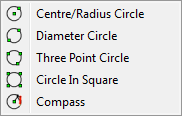There are five different types of circles available in FX Draw.  Each of the circle figures has been created to solve a different drawing problem.  What makes them different is how the circle's control points are used to define the circle.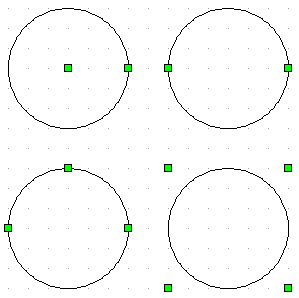When you draw a circle it will be drawn with a default centre mark.  You have a choice of seven centre marks and these can be changed by right clicking on the circle.  These are the same marks that you can choose as points for the point tool.

Circles can be filled using the fill palette (see Setting Fill Type).

The centre mark for the circle can be set as a default.

When you are drawing diagrams including circles, your choice of circle tool can make a large difference to the ease with which you will draw your diagram.

Available Parameters for Circles

\$centre

\$diameter

\$circumference

\$area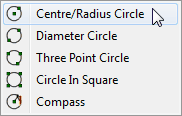The centre & radius circle is defined by the centre of the circle and any point on the circumference.  This is the circle tool to use if you know the location of the centre of the circle.

To draw a centre & radius circle, you mark the centre of the circle with your initial click and the point on the circumference when you release the button.  Your Centre & Radius circle could extend beyond the bounds of the drawing area.  If this occurs, FX Draw will draw as much of the circle as possible.

# Diameter Circle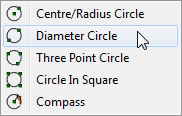When you are drawing a diameter circle, the two points you define will form a diameter of the circle

To draw a diameter circle, you click and hold the left mouse button – move the mouse to the other end of the diameter and release the mouse button.

# Three Point Circle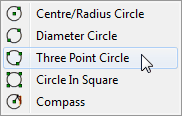Three-point circles are defined by ANY three points on their circumference.  They are ideal for drawing, for example, the circum-circle of a triangle.

To draw a three point circle, just click once on each of the three points.Video - Three Point Circles

# Circle in a Square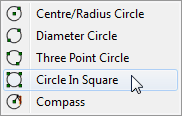The four corner circle will draw a circle inscribed in a square region defined by four control points.

To draw a four corner circle, you click and hold the left mouse button – move the mouse to the diagonally opposite corner and release the mouse button.

# Compass Circle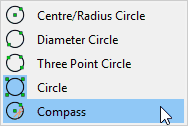A compass circle is defined by a centre point and a pre-defined radius. Every time you click your mouse, you will draw a circle with its centre on the point you click.

When you change to the compass circle tool, the toolbar will change and give you the option to explicitly set the radius of the circles you are drawing.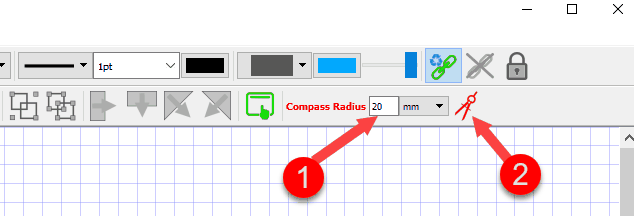Enter the radius you want at (1).

Sometimes you will want to measure a radius on the diagram, rather than typing it explicitly. For example, in this diagram, I might need to make the compass radius equal to the length of AB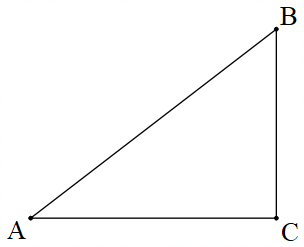To measure this radius, I need to press the Set Radius On Diagram button (2). When this button is pressed, the next compass circle will be drawn in the same way as a normal circle and the radius will be automatically entered as the Compass Radius (1). This allows you to use the compass circle to do geometric constructions.

Once a radius is set, either explicitly or on the diagram, every subsequent circle will be drawn with the same radius. This allows you to quickly create diagrams like this.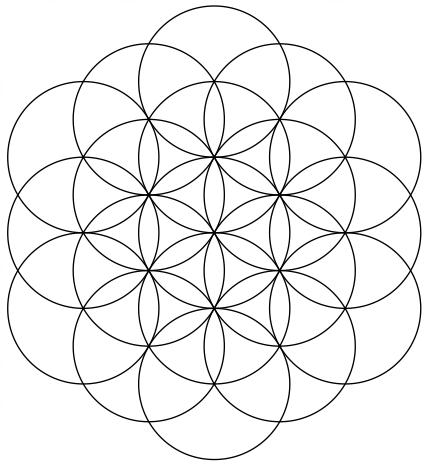We strongly recommend that you view the following video on how to use the compass like tools.FX Draw - How To Use The New Compass Tools